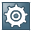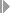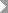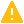###Posting Depreciation

Every asset transaction in Asset Accounting immediately causes a change of the forecasted depreciation. However, an asset transaction does not immediately cause an update of the depreciation and value adjustment accounts for the financial statements. The planned depreciation is posted directly to Financial Accounting when you run the periodic depreciation posting run. This posting run posts the planned depreciation for each posting level for each asset as a lump sum amount.Note

During the posting of depreciation, the system does not create documents for each asset. It creates collective documents instead.

End of the note.

#### Prerequisites

You can choose both the posting cycle and the additional account assignment levels (such as, cost center or order; see the documentation for additional account assignment) for the depreciation posting run for each company code and depreciation area.

To do so, perform the following activities in Customizing for Asset Accounting:

•Integration with General Ledger AccountingPost Depreciation to General Ledger AccountingSpecify Intervals and Posting Rules•Integration with General Ledger AccountingPost Depreciation to General Ledger AccountingAdditional Account Assignment ObjectsYou can enter the accounts to be posted for each depreciation area in each of the account determinations (see the documentation for account determination).

#### Features

The calculation and scheduling of depreciation, interest and revaluation are automatically controlled by keys in the system, or you can control them manually using a special posting transaction. In both cases, planned values from Asset Accounting have to be periodically posted to the corresponding asset and expense accounts of the general ledger. In addition to the various depreciation types, interest and revaluation, the system also posts the allocation and write-off of special reserves during this posting run.Note

End of the note.
##### Performance

It is difficult to provide precise information on performance of the depreciation posting run, since it is strongly dependent on your system configuration. However, you can use the figures below for a rough estimate:

• For the calculation of depreciation, 16000 assets per hour is possible.

• Since only collective documents are created, the number of documents to be posted in a depreciation posting run depends on the number of G/L accounts to be posted, and on the number of combinations of account assignments and account determinations.Caution

Keep in mind that the update run of the depreciation posting run can only be started in the background.

End of the caution.